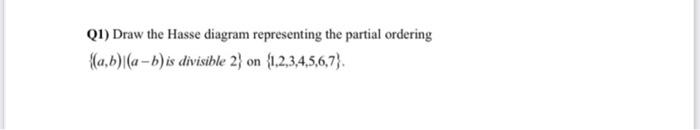Home / Expert Answers / Other Math / q1-draw-the-hasse-diagram-representing-the-partial-ordering-a-b-mid-a-b-is-divisible-pa364

# (Solved): Q1) Draw the Hasse diagram representing the partial ordering \( \{(a, b) \mid(a-b) \) is divisible ...Q1) Draw the Hasse diagram representing the partial ordering \( \{(a, b) \mid(a-b) \) is divisible 2\( \} \) on \( \{1,2,3,4,5,6,7\} \). 2. ask the user to type their name and then tell them how many vowels are in their name. use for

We have an Answer from Expert